Working in the oil field and loving to share knowledge.

## Calculate Annular CapacityAnnular capacity  is volume of fluid between two diameter of cylindrical objects per length or length per volume. This article demonstrates you how to calculate annular capacity between casing or hole and drill pipe, tubing, or casing. There are several formulas as shown below to calculate annular capacity depending on unit of annular capacity required.Note: Dh is bigger ID and Dp is smaller OD. The examples below will show the Dh as hole size and Dp is drill pipe OD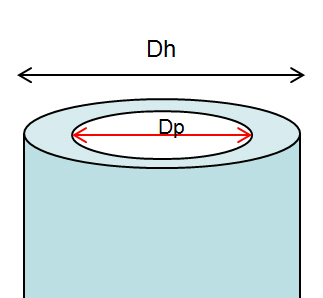## a) Calculate annular capacity in bbl/ft

Annular capacity in bbl/ft =  (Dh2 – Dp2) ÷1029.4

Where;
Dh in inch
Dp in inch

Example: Hole size (Dh) = 6-1/8 in.
Drill pipe OD (Dp) = 3.5  in.
Annular capacity in bbl/ft = (6.1252 – 3.5 2) ÷1029.4
Annular capacity = 0.0245 bbl/ft

## b) Calculate annular capacity in gal/ft

Annular capacity in gal/ft = (Dh2 – Dp2) ÷ 24.51

Where;
Dh in inch
Dp in inch

Example:
Hole size (Dh) = 6-1/8 in.
Drill pipe OD (Dp) = 3.5  in.
Annular capacity in gal/ft = (6.125 2 – 3.52) ÷24.51
Annular capacity = 1.031 gal/ft

## c) Calculate annular capacity in cu-meter/meter (m3/m)

Annular capacity in m3/m =  (Dh2 – Dp2) ÷1,273,240

Where;
Dh in mm
Dp in mm

Example:
Hole size (Dh) = 155.56 mm.
Drill pipe OD (Dp) = 88.90  mm.
Annular capacity in m3/m = (155.562 – 88.90 2) ÷1,273,240
Annular capacity = 0.0128 m3/m

## d) Calculate annular capacity in litre/meter (l/m)

Annular capacity in m3/m =  (Dh2 – Dp2) ÷1,273.24

Where;
Dh in mm
Dp in mm

Example:
Hole size (Dh) = 155.56 mm.
Drill pipe OD (Dp) = 88.90  mm.
Annular capacity in m3/mt = (155.562 – 88.90 2) ÷1,273.24
Annular capacity =12.8 l/m

Annular volume can be determined by this following formula;

## Oilfield Unit

Annular volume in bbl  = annular capacity (bbl/ft) x length of annulus (ft)

Example:

Annular capacity = 0.0245 bbl/ft
Length of annulus = 1000 ft
Annular volume = 1000 x 0.0245 = 24.5 bbl.

## Metric Unit

Annular volume in m3 = annular capacity (m3/m) x length of annulus (m)

Example:

Annular capacity = 0.0128 m3/m
Length of annulus = 330 m
Annular volume = 330 x 0.0128 = 4.224 m3.

** Please remember that if you have several annular profile, you must calculate volume based on each annular profile in order to get total annular volume.This is 2020 update with Metric Unit – Please find the Excel sheet for calculating annular capacity.

Ref books:

Lapeyrouse, N.J., 2002. Formulas and calculations for drilling, production and workover, Boston: Gulf Professional publishing.

Bourgoyne, A.J.T., Chenevert , M.E. & Millheim, K.K., 1986. SPE Textbook Series, Volume 2: Applied Drilling Engineering, Society of Petroleum Engineers.

Mitchell, R.F., Miska, S. & Aadny, B.S., 2011. Fundamentals of drilling engineering, Richardson, TX: Society of Petroleum Engineers.

## Calculate Equivalent Circulation Density (ECD) with complex engineering equations

These formulas below are used for complex calculation for annular pressure loss and equivalent circulating density. I think this calculation will give you more accurate result than a simple equation. Please follow the following steps how to calculate annular pressure loss and ECD.

1. Determine n: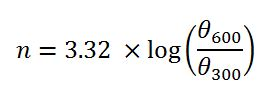2. Determine K: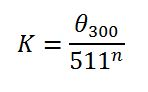3. Determine annular velocity (v) in ft/min: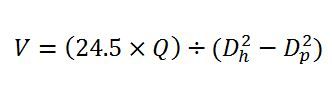4. Determine critical velocity (Vc) in ft/min: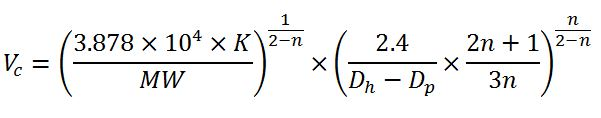5. Pressure loss for laminar flow (Ps), psi:6. Pressure loss for turbulent flow (Ps), psi:7. Determine equivalent circulating density (ECD), ppg: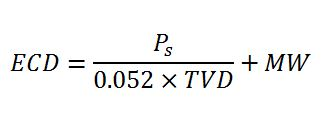Abbreviation meaning

θ300: viscometer dial reading at 300 rpm
θ600: viscometer dial reading at 600 rpm
Q: Flow rate in gpm
Dh: Diameter of hole
Dp: Diameter of drill pipe, drill collar or BHA in ft
v: annular velocity in ft/min
L: length of drill pipe, drill collar or BHA in ft
MW: Mud Weight
PV: Plastic viscosity

Example: Equivalent circulating density (ECD) in ppg by using following data:

Mud weight = 9.5 ppg
θ300 = 40
θ600 = 60
Plastic viscosity = 20 cps
Circulation rate = 650 gpm
Hole diameter = 8.5 in.
Drill collar OD = 6.75 in.
Drill pipe OD = 5.0 in
Drill collar length = 600 ft
Drill pipe length = 10,000 ft
True vertical depth = 9,000 ft

1. Determine n: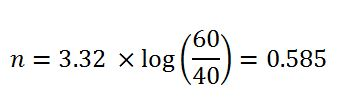2. Determine K: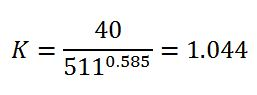3. Determine annular velocity (v) in ft/min around drill pipe: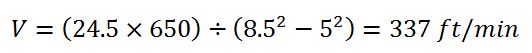4. Determine critical velocity (Vc) in ft/min around drill pipe: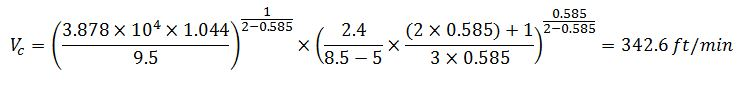The annular velocity around drill pipe is less than the critical velocity around drill pipe so this is laminar flow. The equation #5 (for laminar flow) must be applied in this case.

Pressure loss for turbulent flow (Ps), psi:5. Determine annular velocity (v) in ft/min around drill collar: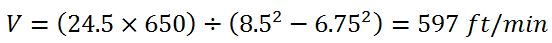6. Determine critical velocity (Vc) in ft/min around drill collar: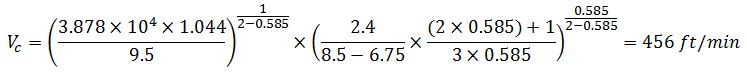The annular velocity around drill collar is more than the critical velocity around drill collar so this is turbulent flow. The equation #6 (for turbulent flow) must be applied in this case.

Pressure loss for laminar flow (Ps), psi:Total annular pressure loss = annular pressure loss around drill pipe + annular pressure loss around drill collar

Ps=271.3+81.5 = 352.8psi

7. Determine equivalent circulating density (ECD), ppg:Please find the Excel sheet for calculating ECD (engineering calculation)

Ref books: Lapeyrouse, N.J., 2002. Formulas and calculations for drilling, production and workover, Boston: Gulf Professional publishing.

Bourgoyne, A.J.T., Chenevert , M.E. & Millheim, K.K., 1986. SPE Textbook Series, Volume 2: Applied Drilling Engineering, Society of Petroleum Engineers.

Mitchell, R.F., Miska, S. & Aadny, B.S., 2011. Fundamentals of drilling engineering, Richardson, TX: Society of Petroleum Engineers.

## Calculate Annular Pressure LossFrom the previous articles, Equivalent Circulating Density (ECD) in ppg, you may want to know how to determine annular pressure loss in order to calculate Equivalent Circulating Density (ECD) in ppg.

So use the following formula to calculate annular pressure loss. This formula will roughly give you idea about how much annular pressure loss you will encounter. For this 2021 update, we’ve added the formula in both oilfield and metric unit.

## Oilfield Unit

P= [(1.4327 × 10-7) × MW × L × V2] ÷ (Dh – Dp)

P = annular pressure losses, psi

MW = mud weight in ppg

L = length of annular in ft

V = annular velocity in ft/min

Dh = hole or casing ID in inch

Dp = drill pipe or drill collar OD in inch

Example:

Mud weight = 13.0 ppg

Length = 8000 ft

Circulation rate = 320 gpm

Hole size = 6.5 in.

Drill pipe OD = 4.0 in.

Determine annular velocity, ft/mm: v = (24.5 x 320) ÷ (6.52 – 4.02)

v = 299 ft/min

Determine annular pressure losses, psi: P = [(1.4327 × 10-7× 13.0 × 8000 × 2992] ÷ (6.5 – 4.0)

P = 531.65 psi

## Metric Unit

P= [(7.39 × 10-6) × MW × L × V2] ÷ (Dh – Dp)

P = annular pressure losses, KPa

MW = mud weight in kg/m³

L = length of annular in m

V = annular velocity in m/min

Dh = hole or casing ID in mm

Dp = drill pipe or drill collar OD in mm

Example:

Mud weight = 1,560 kg/m³

Length = 2,400 m

Circulation rate = 1,200 l/m

Hole size = 165 mm

Drill pipe OD = 100 mm

Determine annular velocity, ft/mm: v = (1,000 × 1,200) ÷ (π × (1652 – 1002) ÷ 4)

v = 88.7 m/min

Determine annular pressure losses, psi: P = [(7.39 × 10-6× 1560 × 2400 × 88.72] ÷ (165 – 100)

P = 3,349 KPa

PS, This is the estimation for annular pressure. For more accurate, you may need to consult to a drilling fluid company to perform simulation since they have advanced software that can account for many parameters ie cutting loading, mud rheology, etc.Ref books:

Lapeyrouse, N.J., 2002. Formulas and calculations for drilling, production and workover, Boston: Gulf Professional publishing.

Bourgoyne, A.J.T., Chenevert , M.E. & Millheim, K.K., 1986. SPE Textbook Series, Volume 2: Applied Drilling Engineering, Society of Petroleum Engineers.

Mitchell, R.F., Miska, S. & Aadny, B.S., 2011. Fundamentals of drilling engineering, Richardson, TX: Society of Petroleum Engineers.

## Equivalent Circulating Density (ECD) in both Oilfield and Metric UnitEquivalent Circulating Density (ECD) is the effective density that combines current mud density and annular pressure drop. ECD is vital for drilling engineering since it limits how depth of each section to be drilled and leads to losses.The equivalent circulating density formula is shown below;

## Oilfield Unit

Equivalent Circulating Density (ECD) = (Annular Pressure Loss ÷ 0.052 ÷ True Vertical Depth (TVD)) + (Current Mud Weight)

Equivalent Circulating Density (ECD) in ppg

Annular Pressure Loss in psi

True Vertical Depth (TVD) in ft

Current Mud Weight in pppg

Example:

Annular pressure loss = 400 psi
True Vertical Depth = 8,000 ft
Current mud weight in ppg = 10 ppg

ECD in ppg =   (400 psi ÷ 0.052 ÷ 8,000 ft) +10.0 ppg

ECD = 11.0 ppg

## Metric Unit

Equivalent Circulating Density (ECD) = (Annular Pressure Loss × 1000 ÷ 9.81÷ True Vertical Depth (TVD) ) + (Current Mud Weight)

Equivalent Circulating Density (ECD) in kg/m3

Annular Pressure Loss in KPa

True Vertical Depth (TVD) in m

Current Mud Weight in kg/m3

Example:

Annular pressure loss = 2760 KPa
True Vertical Depth = 2,440 m
Current mud weight in ppg = 1200  kg/m3

ECD in ppg =  (2,760 Kpa  × 1000 ÷ 9.81÷ 2,440 m )  + 1,200 kg/m3

ECD = 1,315 kg/m3

Please find the Excel sheet to calculate Equivalent Circulating Density (ECD)Ref books:

Lapeyrouse, N.J., 2002. Formulas and calculations for drilling, production and workover, Boston: Gulf Professional publishing.

Bourgoyne, A.J.T., Chenevert , M.E. & Millheim, K.K., 1986. SPE Textbook Series, Volume 2: Applied Drilling Engineering, Society of Petroleum Engineers.

Mitchell, R.F., Miska, S. & Aadny, B.S., 2011. Fundamentals of drilling engineering, Richardson, TX: Society of Petroleum Engineer

## Annular Velocity (AV) CalculationAnnular Velocity (AV) is how fast of fluid flow in  the annulus while pumping.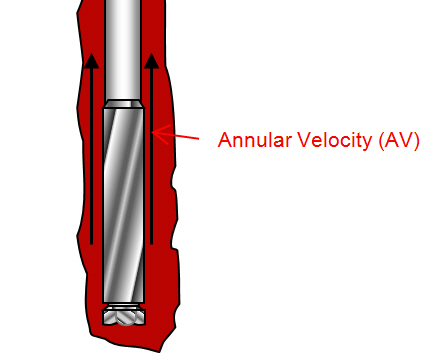Three main factors affecting annular velocity are size of hole (bigger ID), size of drill pipe (smaller OD) and pump rate. This post will show you how to calculate annular velocity in feet per minute with different formulas.

# Annular Velocity Formula

## Oilfield Unit

Formula#1: Annular velocity (AV) in ft/min

Annular velocity = Flow Rate÷ Annular Capacity

where;

Annular velocity in ft/min

Flow rate in bbl/min

Annular capacity in bbl/ft

Example:
Flow rate = 10 bbl/min
Annular capacity = 0.13 bbl/ft
AV = 10 bbl/min ÷ 0.13 bbl/ft
AV = 76.92 ft/min

Formula#2: Annular velocity (AV) in ft/min

Annular velocity = (24.5 × Flow Rate) ÷ (Dh2 – Dp2)

where;
Annular velocity in ft/min
Flow Rate in gpm
Dh = inside diameter of casing or hole size in inch
Dp = outside diameter of pipe, tubing or collars in inch

Example:
Flow rate = 800 gpm
Hole size = 10 in.
Drill pipe OD = 5 in.
AV = (24.5 × 800) ÷ (102 – 52)
AV = 261 ft/mim

Formula#3: Annular velocity (AV) in ft/min

Annular Velocity  = Flow Rate × 1029.4÷ (Dh2 – Dp2)

Where;
Annular Velocity in ft/min
Flow Rate in bbl/min

Example:
Flow rate = 13 bbl/min
Hole size = 10 in.
Drill pipe OD = 5 in.
Annular Velocity = 13 bbl/min × 1029.4 ÷ (102 – 52)
Annular Velocity = 178.43 ft/min

## Metric Unit

Formula#1: Annular velocity (AV) in m/min

Annular velocity = Flow Rate÷ Annular Capacity

where;
Annular velocity in m/min
Flow rate in liters/min
Annular capacity in liters/m

Example:
Flow rate = 1600 liters/min
Annular capacity = 68 liters/m
Annular Velocity = 1600 liters/min÷ 68 liters/m
Annular Velocity = 23.5 m/min

Formula#2: Annular velocity (AV) in m/min

Annular velocity = (4000× Flow Rate) ÷ (π×(Dh2 – Dp2))

where;
Annular velocity in m/min
Flow Rate in liters/min
Dh = inside diameter of casing or hole size in mm
Dp = outside diameter of pipe, tubing or collars in mm

Example:
Flow rate = 3000 liters/min
Hole size = 254 mm
Drill pipe OD = 127 mm
Annular Velocity = (4000 × 3000) ÷ (π×(2542 – 1272))
Annular Velocity = 78.9 m/min

Formula#3: Annular velocity (AV) in m/min

Annular Velocity  = (Flow Rate × 4 ×106 ) ÷ (π×(Dh2 – Dp2))

Where;
Annular Velocity in m/min
Flow Rate in m³/min
Dh = inside diameter of casing or hole size in mm
Dp = outside diameter of pipe, tubing or collars in mm

Example:
Flow rate = 2 m³/min
Hole size = 254 mm
Drill pipe OD = 127 mm
AV = (2 m³/min 4 ×106 ) ÷ (π×(102 – 52))
AV = 52.6 m/min

# Flow Rate to Achieve Require Annular Velocity

## Oilfield Unit

Flow rate required in gpm = (Annular Velocity ) × (Dh2 – DP2) ÷ 24.5

Where;
Flow rate in gpm
Annular Velocity in ft/min
Dh = inside diameter of casing or hole size in inch
Dp = outside diameter of pipe, tubing or collars in inch

Example:
Desired annular velocity = 120 ft/mm
Hole size = 10 in
Drill pipe OD = 5 in.
Flow rate required in gpm = 120 × (102– 52) ÷ 24.5
Flow rate required in gpm = 367.4 gpm

## Metric Unit

Flow rate required in gpm = (Annular Velocity × π) × (Dh2 – DP2) ÷ 4000

Where;
Flow rate in liters/min
Annular Velocity in m/min
Dh = inside diameter of casing or hole size in mm
Dp = outside diameter of pipe, tubing or collars in mm

Example:
Desired annular velocity = 37 m/min
Hole size = 254 mm
Drill pipe OD = 127 mm
Flow rate required  = (37 × π) × (2542– 1272) ÷ 4,000
Flow rate required  = 1,406 liters/min

# Stroke per Minute to Achieve Require Annular Velocity

## Oilfield Unit

SPM = (Annular Velocity × Annular Capacity) ÷ Pump Output

Where;
Annular Velocity in ft/min
Annular Capacity in bbl/ft
Pump output in bbl/stk

Example:
Desired annular velocity in ft/min = 120 ft/min
Dh = 12-1/4 in.
Dp = 4-1/2 in.
Annular capacity = 0.1261 bbl/ft
Pump output = 0.136 bbl/stk
SPM = (120 ft/min× 0.1261 bbl/ft) ÷ 0.136 bbl/stk
SPM = 111.3 spm

## Metric Unit

SPM = (Annular Velocity × Annular Capacity) ÷ Pump Output

Where;
Annular Velocity in m/min
Annular Capacity in liters/m
Pump output in liters/stk

Example:
Desired annular velocity in m/min = 46 m/min
Hole size = 254 mm
Drill pipe OD = 127 mm
Annular capacity = 0.0380 m³/m
Pump output = 0.0156 m³/stk
SPM = (46 m/min × 0.0380 bbl/ft) ÷ 0.0156 m³/stk
SPM = 112 spm

Please find the Excel sheet  for calculating annular velocityRef books: Lapeyrouse, N.J., 2002. Formulas and calculations for drilling, production and workover, Boston: Gulf Professional publishing.

Bourgoyne, A.J.T., Chenevert , M.E. & Millheim, K.K., 1986. SPE Textbook Series, Volume 2: Applied Drilling Engineering, Society of Petroleum Engineers.

Mitchell, R.F., Miska, S. & Aadny, B.S., 2011. Fundamentals of drilling engineering, Richardson, TX: Society of Petroleum Engineers.